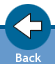Glossary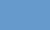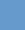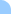Amplitude: The maximum displacement from the state of equilibrium during vibration. In the case of waves within wave-propagation, it is the maximum displacement from the state of equilibrium of the oscillating particles. Symbol: A Physical unit: 1 metre (1 m)Fixed end: the edge of the wave space, where the particles of the elastic medium cannot oscillate.   Free (Open) end: the edge of the wave space, where the particles of the elastic medium can oscillate freely.   Frequency: It shows the number of oscillations performed in one second. Symbol: f Physical unit: 1 Hertz (1 Hz = 1/second)   Interference: When two or more waves meet, in the meeting area the amplitudes of the oscillations of the waves are summed so that the algebraic sign is to be taken into consideration. For example, two antinodes can create a bigger antinode, while a node and an antinode can flatten the wave.   Phase: Such a state of the body carrying out the harmonic oscillation, which is determined by the part of the periodical movement in which the body is at a given moment.   Propagation speed: The propagation speed of the oscillation phase is defined as the propagation (phase)speed of the wave. Symbol: c Physical unit: 1 metre/second (1 m/s)   Wavelength:The particles at a big distance from the wave-source start their oscillatory movement later than those closer to the source. Consequently, they are in a different phase of oscillation at a given moment. The wavelength is the distance between the two closest adjacent points in the same phase. Symbol: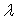Physical unit: 1 metre (1 m)